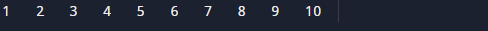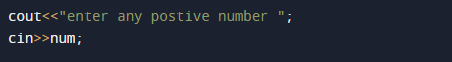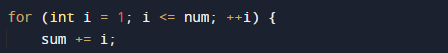C++ Program to Calculate Sum of Natural Numbers

In this tutorial you will learn about the C++ Program to Calculate Sum of Natural Numbers and its application with practical example.

In this tutorial, we will learn to create a C++ program that  will find Sum of N Natural Numbers  using C++ programming.

Prerequisites

Before starting with this tutorial we assume that you are best aware of the following C programming topics:

• Operators.
• looping statements.
• Basic input/output.
• Basic c++ programming.

What Is Natural  Number?

Simply all the counting numbers {…-3,-2-1,0,1, 2, 3, …} are commonly called natural numbers.Natural numbers As such, it is a whole, non-negative are all number 1, 2, 3, 4,5,6,7,8,9,10… They are usually counting  number and they will continue to infinity.while Whole number are all natural numbers including 0.

C++ Program to Calculate Sum of Natural Numbers

Firstly we declare required header file and variable and also initiates required values in variable. Next we take value from user at run time and then after we will find the sum of all natural number to given value.

OutputIn the above program, we have first declared and initialized a set variables required in the program.

• i= for iteration of loop.
• num=to take value from user.
• sum = for calculating sum of natural number.

In this program first we take a value from user,After that in this Program we add all natural number from 1 to 10.

• The first we will create  an integer variable i,num and sum.
• then, we use For Loop to iterate between i=1 to i<=10 and• Within the For loop, we add all i values in variable sum. sum+=i.
• In our  program we calculate sum of natural value from 1- 10.
• At last we print the sum of 10 natural values.that’s all fro this post thank-you.

In this tutorial we have learn about the C++ Program to Calculate Sum of Natural Numbers and its application with practical example. I hope you will like this tutorial.